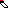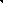# MINLPLib

### A Library of Mixed-Integer and Continuous Nonlinear Programming Instances

#### Instance: alan

 Formatsⓘ ams gms lp mod nl osil pip Primal Boundsⓘ 2.92500000 p1 ( gdx sol ) (infeas: 0) Dual Boundsⓘ 2.92500000 (ALPHAECP)2.92500000 (ANTIGONE)2.92500000 (BARON)2.92500000 (BONMIN)2.92500000 (COUENNE)2.92500000 (LINDO)2.92500000 (SCIP) Referencesⓘ Manne, Alan S, GAMS/MINOS: Three examples, Tech. Rep., Department of Operations Research, Stanford University, 1986. Sourceⓘ GAMS Model Library model alan Applicationⓘ Portfolio Optimization Added to libraryⓘ 01 May 2001 Problem typeⓘ MBQP #Variablesⓘ 8 #Binary Variablesⓘ 4 #Integer Variablesⓘ 0 #Nonlinear Variablesⓘ 3 #Nonlinear Binary Variablesⓘ 0 #Nonlinear Integer Variablesⓘ 0 Objective Senseⓘ min Objective typeⓘ quadratic Objective curvatureⓘ convex #Nonzeros in Objectiveⓘ 3 #Nonlinear Nonzeros in Objectiveⓘ 3 #Constraintsⓘ 7 #Linear Constraintsⓘ 7 #Quadratic Constraintsⓘ 0 #Polynomial Constraintsⓘ 0 #Signomial Constraintsⓘ 0 #General Nonlinear Constraintsⓘ 0 Operands in Gen. Nonlin. Functionsⓘ Constraints curvatureⓘ linear #Nonzeros in Jacobianⓘ 20 #Nonlinear Nonzeros in Jacobianⓘ 0 #Nonzeros in (Upper-Left) Hessian of Lagrangianⓘ 9 #Nonzeros in Diagonal of Hessian of Lagrangianⓘ 3 #Blocks in Hessian of Lagrangianⓘ 1 Minimal blocksize in Hessian of Lagrangianⓘ 3 Maximal blocksize in Hessian of Lagrangianⓘ 3 Average blocksize in Hessian of Lagrangianⓘ 3.0 #Semicontinuitiesⓘ 0 #Nonlinear Semicontinuitiesⓘ 0 #SOS type 1ⓘ 0 #SOS type 2ⓘ 0 Infeasibility of initial pointⓘ 0.5048 Sparsity JacobianⓘSparsity Hessian of Lagrangianⓘ```\$offlisting
*
*  Equation counts
*      Total        E        G        L        N        X        C        B
*          8        3        0        5        0        0        0        0
*
*  Variable counts
*                   x        b        i      s1s      s2s       sc       si
*      Total     cont   binary  integer     sos1     sos2    scont     sint
*          9        5        4        0        0        0        0        0
*  FX      0
*
*  Nonzero counts
*      Total    const       NL      DLL
*         24       21        3        0
*
*  Solve m using MINLP minimizing objvar;

Variables  x1,x2,x3,x4,objvar,b6,b7,b8,b9;

Positive Variables  x1,x2,x3,x4;

Binary Variables  b6,b7,b8,b9;

Equations  e1,e2,e3,e4,e5,e6,e7,e8;

e1..    x1 + x2 + x3 + x4 =E= 1;

e2..    8*x1 + 9*x2 + 12*x3 + 7*x4 =E= 10;

e3.. x1*(4*x1 + 3*x2 - x3) + x2*(3*x1 + 6*x2 + x3) + x3*(x2 - x1 + 10*x3)
- objvar =E= 0;

e4..    x1 - b6 =L= 0;

e5..    x2 - b7 =L= 0;

e6..    x3 - b8 =L= 0;

e7..    x4 - b9 =L= 0;

e8..    b6 + b7 + b8 + b9 =L= 3;

* set non-default levels
x1.l = 0.302884615384618;
x2.l = 0.0865384615384593;
x3.l = 0.504807692307693;
x4.l = 0.10576923076923;
objvar.l = 2.89903846153846;

* set non-default marginals
e1.m = 1;
e2.m = 1;
e3.m = 1;
x1.m = 1;
x2.m = 1;

Model m / all /;

m.limrow=0; m.limcol=0;
m.tolproj=0.0;

\$if NOT '%gams.u1%' == '' \$include '%gams.u1%'

\$if not set MINLP \$set MINLP MINLP
Solve m using %MINLP% minimizing objvar;

```

Last updated: 2019-02-14 Git hash: a71254dc